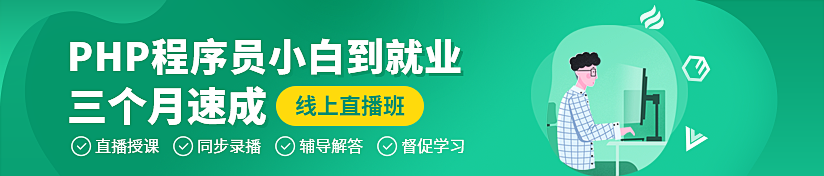# JavaScript详细解析之作用域链【相关推荐：JavaScript视频教程web前端

## 1. 作用域是什么？

• `作用域首先是一套规则`
• `该规则的用处是用来存储变量，以及如何有限制的获取变量。`

## 2. 词法作用域与动态作用域

```var a = 1function foo() {  console.log(a)
}function bar(b) {  var a = 2
console.log(a)  foo()  function baz() {      console.log(b)
}  return baz
}var c = bar(a)c()```

bar先调用并传入变量a， 在第一个输出变量a值时， 完全获取到变量a因而输出2。在调用foo函数时， 由于自身作用域没有变量a， 则会`从自身被调用的位置的作用域`去往上查找，则此时为函数bar的作用域，因而输出的a值为2。

c函数调用也就是baz函数调用时，也同样是自身不存在变量b，去寻找`自身被调用的位置的作用域`，也就是全局作用域，全局作用域中同样未定义过变量b， 则直接报错。

## 3. 作用域的分类

• `静态作用域`
• `动态作用域`

• `全局作用域`
• `函数作用域`
• `块级作用域`

### 3.2 函数作用域

• 可以存在一个更小的范围存放自身内部的变量和函数，外部无法访问
• 由于外部无法访问，所以相当于隐藏了内部细节，仅提供输入和输出，符合最小暴露原则
• 同时不同的函数作用域可以各自命名相同的变量和函数，而不产生命名冲突
• 函数作用域可以嵌套函数作用域，就像俄罗斯套娃一样可以一层套一层，最终形成了作用域链

```var name = 'xavier'function foo() {  var name = 'parker'
var age = 18

function bar() {    var name = 'coin'
return age
}  return bar()
}foo()console.log(age) // 报错```

### 3.3 块级作用域

```let obj = {  a: 1,   // 这个区域不叫做块级作用域}

if (true) {  // 这个区域属于块级作用域
var foo = 1
let bar = 2}console.log(foo)  // 1console.log(bar)  // 报错```

## 4. 执行上下文和作用域的关系

• 变量对象
• 作用域链
• this

```var a = 'a'function foo() {    let b = 'b'
console.log(c)
}if (true) {    let a = 'c'
var c = 'c'
console.log(a)
}foo()console.log(a)```## 6. 一些练习

### 6.1 自己设计一道简单的练习题

```var a = 10let b = 20const c = {  d: 30}function foo() {  console.log(a)  let e = 50
return b + e
a = 40}function bar() {  console.log(f)  var f = 60
let a = 70
console.log(f)  return a + c.d}if (a <= 30) {  console.log(a)  let b = foo()  console.log(b)
}

console.log(b)
c.d = bar()console.log(a)console.log(c.d)```

【相关推荐：JavaScript视频教程web前端1/1